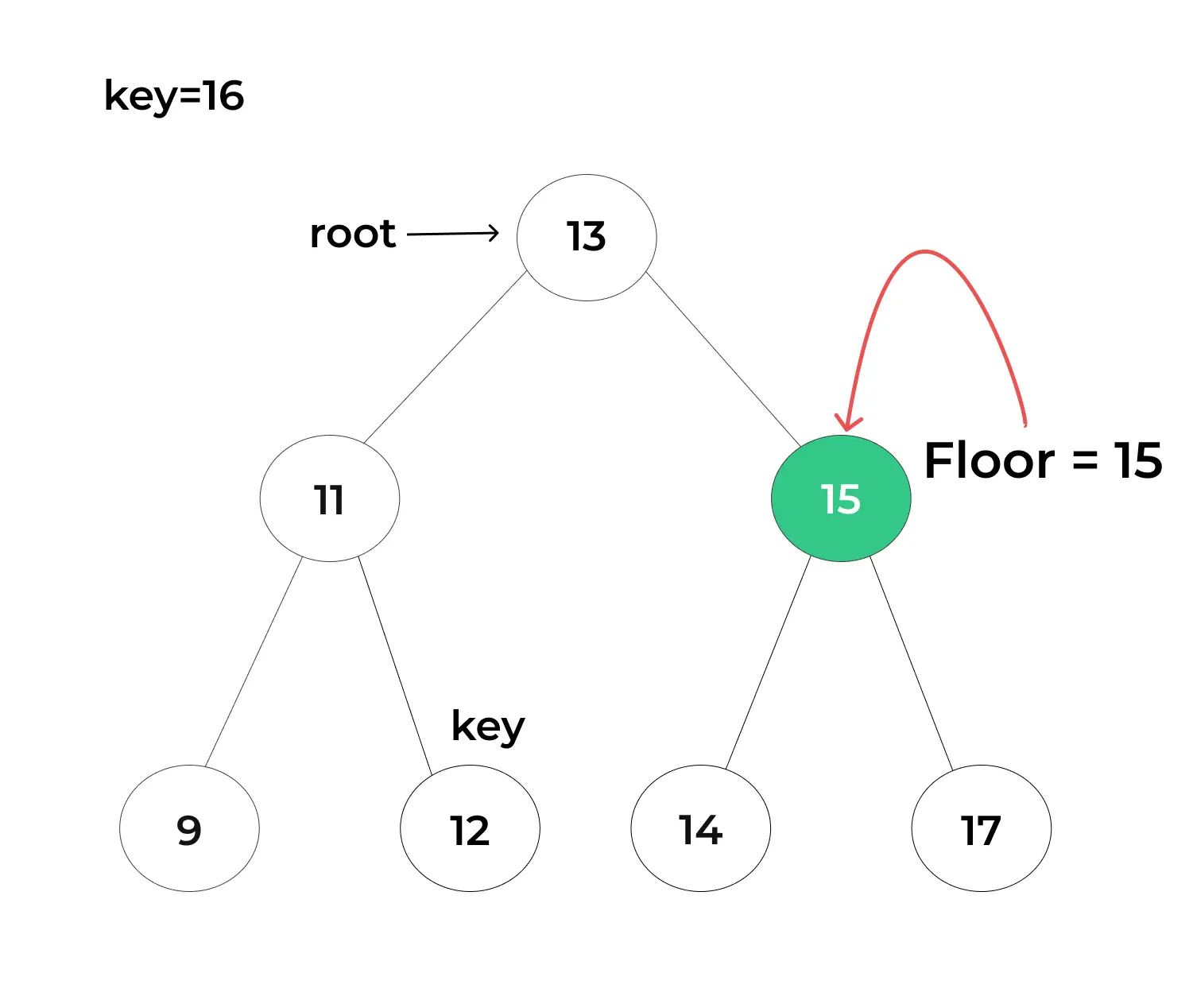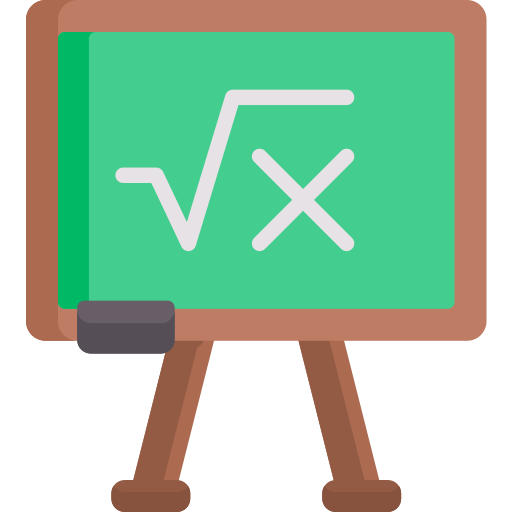# Floor in Binary Search Tree## Exploring the Floor in BST (Binary Search Tree)

When it comes to navigating the intricate world of Binary Search Trees (BSTs), understanding the concept of “floor” is akin to discovering a hidden gem. From the intricacies of the floor operation to its real-world implications, join us in unraveling the magic of BSTs and their floors.

Let’s understand the concept of Floor in BST with its code, complexity and real-world applications.

## What is Floor in BST?

In the context of a Binary Search Tree (BST), the “floor” refers to a fundamental concept used to find the largest element in the BST that is less than or equal to a specific target value. In other words, it’s the greatest key in the tree that is not greater than the given value.

## Example :The above example demonstrate the value of Floor value to be “15”(closest value to the target value) as the target value is “16”.

## Algorithm for finding Floor in BST:

1. Begin the search at the root node of the tree.
2. If the data in the root node is equal to the target key, then the floor value of the key is equivalent to the root’s data.
3. Alternatively, if the data in the root node is greater than the target key, this indicates that the floor value of the key resides in the left subtree.
4. Conversely, if the floor value is not in the left subtree, it may be found in the right subtree, but only if there exists a value less than or equal to the target key in that subtree.
5. If no such value is found in the right subtree, then the root node itself is the floor value for the key.
##### Code for Floor in BST (Binary Search Tree)
```class TreeNode:
def __init__(self, key):
self.key = key
self.left = None
self.right = None

def find_floor(root, target):
floor_value = None
current = root

while current:
if target == current.key:
return current.key
elif target < current.key:
current = current.left
else:
floor_value = current.key
current = current.right

return floor_value

if __name__ == "__main__":
root = TreeNode(50)
root.left = TreeNode(30)
root.right = TreeNode(70)
root.left.left = TreeNode(20)
root.left.right = TreeNode(40)
root.right.left = TreeNode(60)
root.right.right = TreeNode(80)

target_value = 45
result = find_floor(root, target_value)

if result is not None:
print("Floor of", target_value, "is", result, ".")
else:
print("No floor found for", target_value, ".")
```

### Output :

```Floor of 45 is 40 .
```
##### Explanation
• This Python code finds the floor of a target value in a Binary Search Tree (BST). The “find_floor” function iteratively traverses the tree, updating the floor candidate as it progresses.
• If an exact match is found, it returns the current node’s key as the floor.
• If the target value is smaller, it moves left; if larger, it moves right.
• The final floor candidate is returned as the largest key less than or equal to the target value.

### Code:

```class TreeNode:
def __init__(self, key):
self.key = key
self.left = None
self.right = None

def find_floor(root, target):
floor_value = None
current = root

while current:
if target == current.key:
return current.key
elif target < current.key:
current = current.left
else:
floor_value = current.key
current = current.right

return floor_value

# Example usage:
if __name__ == "__main__":
root = TreeNode(60)
root.left = TreeNode(30)
root.right = TreeNode(90)
root.left.left = TreeNode(20)
root.left.right = TreeNode(50)
root.right.left = TreeNode(70)
root.right.right = TreeNode(100)

target_value = 55
result = find_floor(root, target_value)

if result is not None:
print("Floor of", target_value, "is", result, ".")
else:
print("No floor found for", target_value, ".")
```

### Output :

```Floor of 55 is 50 .
```
##### Explanation
• In this example, the Python code effectively computes the floor of the target value 55 in a BST.
• The “find_floor” function iterates through the tree, updating the floor candidate as it progresses.
• When an exact match is not found, it traverses left or right depending on the comparison with the current node’s key.
• The final floor candidate, which is the largest key less than or equal to the target value, is reported.
##### Significance of Finding the Floor in BST

The floor operation in a BST is significant for several reasons:

1. Data Retrieval: It allows us to efficiently retrieve the largest key that is less than or equal to a given value in a sorted dataset, which can be helpful in search and retrieval operations.

2. Range Queries: Floor is often used in range queries to find values within a specific range efficiently. It helps identify the starting point of a range.

3. Interpolation: It plays a role in interpolation algorithms where we estimate a value based on surrounding data points.

##### Practical Use cases of Floor

The floor operation finds applications in various domains:

1. Finance: Calculating the floor of stock prices or interest rates can help in financial modeling and risk analysis.

2. Databases: In databases, floor is useful for range queries, indexing, and data retrieval.

3. Gaming: In game development, floor values are essential for physics simulations, collision detection, and character movement.

4. Statistics: It is used in statistical analysis, especially in estimating values within a given range.

5. Geospatial Data: When dealing with geospatial data, the floor operation aids in locating nearby geographic features or points of interest.

##### Complexity Analysis Floor in BST

The time complexity for finding the floor in a Binary Search Tree (BST) can be analyzed as follows:

Time Complexity: O(h), where ‘h’ is the height of the BST.

• The key factor affecting the time complexity is the height of the tree. In the worst-case scenario, when the BST is unbalanced and resembles a linked list, the height can be ‘n’ (the number of nodes in the tree).
• However, in a well-balanced BST (like a perfectly balanced binary search tree or AVL tree), the height is logarithmic with respect to the number of nodes (h = log2(n)). In such cases, the time complexity for finding the floor is O(log n), which represents an efficient and logarithmic time complexity.

Space Complexity :

• The space complexity for finding the floor is O(1) because it does not require additional data structures or recursion that consume extra memory.
##### To wrap it up:

The concept of floor in a Binary Search Tree is a powerful tool for efficiently finding the largest key less than or equal to a given value. Its significance extends to various fields, making it a valuable operation in data retrieval, range queries, and estimation tasks. Understanding how to implement and utilize the floor operation in BSTs can greatly enhance the efficiency of algorithms and applications that rely on sorted data.

### Related Banners

Get PrepInsta Prime & get Access to all 200+ courses offered by PrepInsta in One SubscriptionQuestion 1.

How is the floor different from the ceiling in a BST?

The floor finds the largest key less than or equal to a target value, while the ceiling finds the smallest key greater than or equal to a target value.Question 2.

Can the floor exist even if the target value is not in the BST?

Yes, the floor can exist in the BST even if the target value is not present. It is the largest key in the tree that is less than or equal to the target value.Question 3.

How does the floor operation in a BST differ from linear search in an array?

The floor operation in a BST is more efficient, with a time complexity of O(log n) in a balanced tree, compared to linear search in an array, which has a time complexity of O(n).Question 4.

Is it possible to find the floor in an empty BST?

No, you cannot find the floor in an empty BST because there are no nodes to compare the target value to.

## Get over 200+ course One Subscription

Courses like AI/ML, Cloud Computing, Ethical Hacking, C, C++, Java, Python, DSA (All Languages), Competitive Coding (All Languages), TCS, Infosys, Wipro, Amazon, DBMS, SQL and others